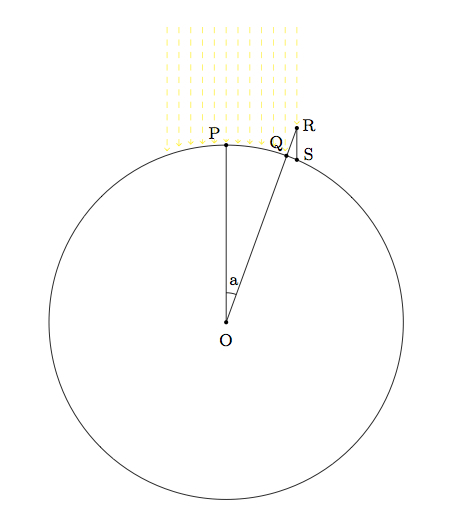# Eratosthenes and the circumference of the earth

Alignments to Content Standards: G-MG.A

The ancient Greek scientist Eratosthenes devised the following experiment for estimating the circumference of the earth, which he assumed to be spherical in shape. Pictured below is the sun's rays hitting two different locations on the surface of the earth. The point $P$, in the picture below, is in the tropics and, at this particular time, the sun's rays are hitting this point perpendicularly. At the point $Q$, the sun's rays meet the earth at an angle which can be measured by finding the length of the shadow cast by an object at point $Q$.1. Assuming the rays of the sun hitting points $P$ and $R$ are parallel as in the picture, explain why angle $POQ$ is congruent to angle $QRS$.
2. For the two locations used by Eratosthenes, the shadow cast by a ten foot pole at location $Q$ was about 1.26 feet. Using this information, find $a$, the measure of angle $POQ$.
3. According to Eratosthenes, the distance from point $P$ to point $Q$ was approximately $2,584,000$ feet. Using this information and the calculation from part (b) what estimate does this give for the circumference of the earth, in both feet and miles?
4. Current estimates for the earth's circumference are about 24,900 miles. Within what percent error from this current value is Eratosthenes' estimate?

## IM Commentary

The picture has been drawn with $a = 20$ because the $7.2$ degree angle which would accurately represent the measurements taken by Eratosthenes was too small to allow space for all of the designated points. Also of course the points $R$ and $S$ are not intended to be ''realistic'' as in practice the height of the object casting a shadow will be very small compared to the circumference of the earth.

The accuracy and simplicity of this experiment are amazing. A wonderful project for students, which would necessarily involve team work with a different school and most likely a school in a different state or region of the country, would be to try to repeat Eratosthenes' experiment. Since the Continental United States does not have any land within the tropics, students would either have to collaborate with a school in Hawaii or alter Eratosthenes' method. What happens to this method if point $P$ is moved to the other side of $Q$?

Teachers may wish to discuss some of the hypotheses behind this method. For example, is it reasonable to assume that the rays of sun reaching points $P$ and $Q$ are parallel? How do you measure the distance from $P$ to $Q$? With GPS systems this is less of an issue today but for Eratosthenes it must have presented a formidable challenge. Teachers may also wish to give more of a historical context to this problem. The two cities where measurements were taken were Syene (modern Aswan in southern Egypt) and Alexandria (also in Egypt) at noon of the summer solstice: this way the sun was directly overhead at this moment at one of the two locations, namely Syene. Since Alexandria is directly north of Syene, Eratosthenes was estimating the polar circumference of the earth which is slightly smaller than the equatorial circumference.

This task is intended mainly for instructional purposes, giving an interesting context for implementing ideas from geometry and trigonometry.

## Solution

1. The rays of the sun hitting the earth are assumed to be parallel: this is a reasonable hypothesis because their point of origin, the sun, is so far away from the earth that they are very close to being parallel. Points $O$ and $P$ are collinear with one ray of the sun while points $R$ and $S$ are collinear with a second ray. So lines $OP$ and $RS$ are parallel. Since segement $OR$ is a transverse of these parallel lines it follows that alternate interior angles $POQ$ and $ORS$ are congruent. Angle $QRS$ is the same as angle $ORS$ so angles $POQ$ and $ORS$ are congruent. This means that angle $ORS$ has measure $a$.
2. The pole may be assumed to make a right angle with the ground so triangle $RQS$ is a right triangle with right angle $RQS$ and hypotenuse $RS$. We are given that $|RQ| = 10$ feet while $|QS| = 1.26$ feet. Using the Pythagorean theorem, we find
\begin{align} |RS|^2 &= |RQ|^2 + |QS|^2\\ &= 10^2 + 1.26^2. \end{align}
Using a calculator we find that $|RS|$ is about $10.08$ feet. So
\begin{align} \frac{|QS|}{|RS|} &\approx \frac{1.26}{10.08} \\ &= 0.125. \end{align}

Now $\frac{|QS|}{|RS|}$ represents the length of the side opposite angle $QRS$ divided by the length of the hypotenuse of triangle $QRS$. This is the sine of the angle $QRS$. With a calculator we can find that angle $QRS$ measures about $7.2$ degrees.

There is a subtlety here which has not been taken into account, namely that the earth is ''curved'' and so the surface of the earth going from $P$ to $Q$ is a small arc rather than a line segment. The circumference of the earth is so large, however, compared to the length of the shadow of the pole, that we may assume that $\overline{PQ}$ is a line segment as is done above.

3. There are 360 degrees around the circle representing the earth and so the 7.2 degrees between $P$ and $Q$ represents $\frac{1}{50}$ of the full circumference. So if the distance from $P$ to $Q$ is estimated at 2,584,000 feet then the full circumference of the earth would be about $$50 \times 2,584,000 \,\,\,\text{ feet} = 129,200,000 \,\,\,\text{ feet}.$$ To convert this to miles we have
\begin{align} 129,200,000 \,\,\,\text{ feet} &= 129,200,000 \,\,\,\text{ feet} \times \frac{1}{5280} \,\,\,\frac{\text{ miles}}{\text{ foot}} \\ &\approx 24,470 \,\,\,\text{ miles}. \end{align}
4. This differs by about 430 miles from the current estimate of the circumference of the earth or about 1.7 percent.